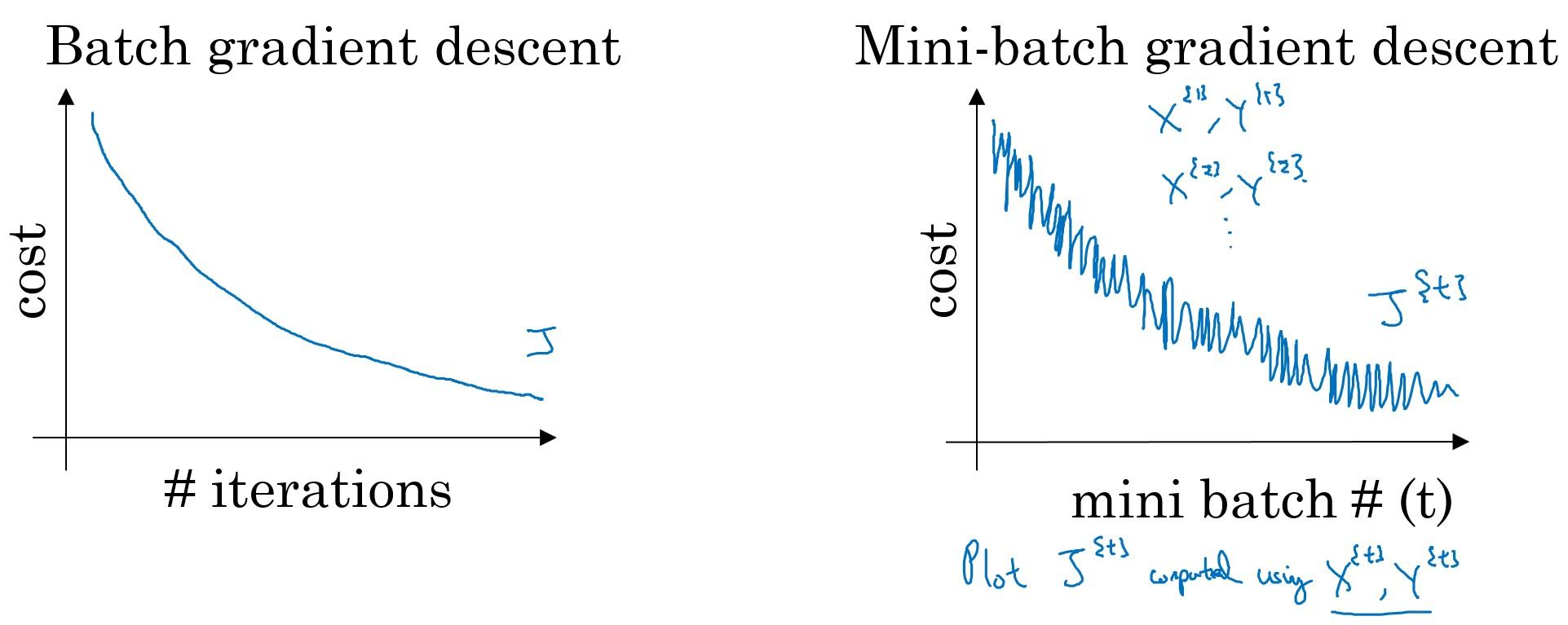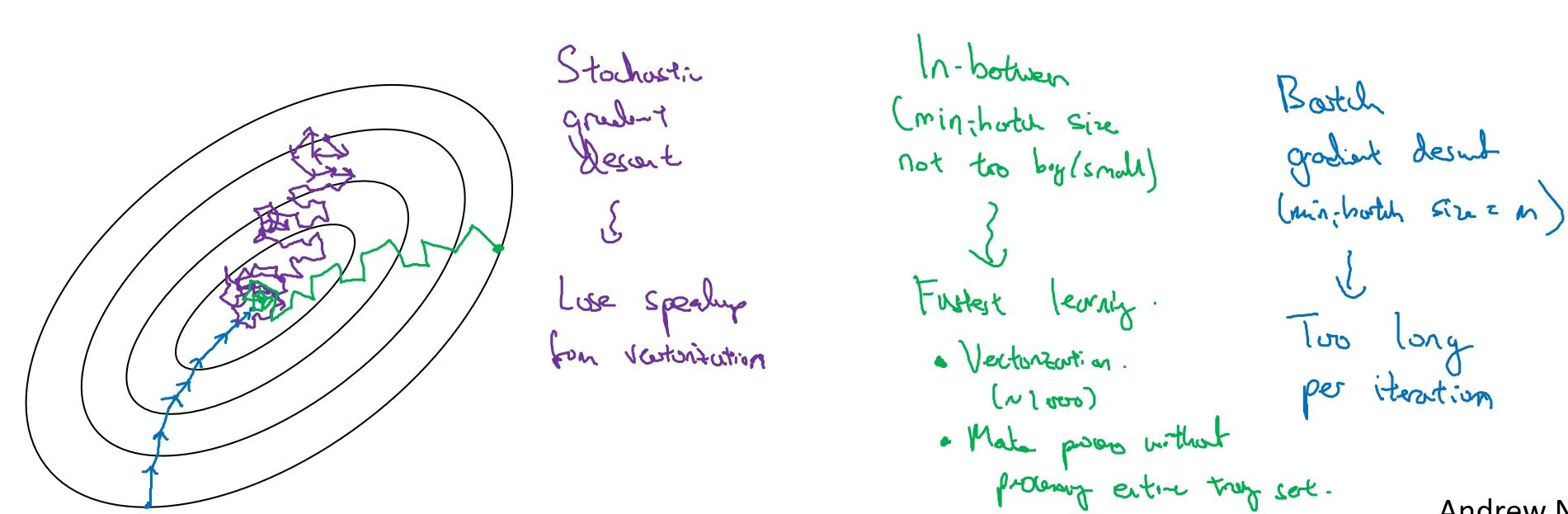# Mini-Batch Gradient Descent and Learning Rate Decay

Categories:

Updated:

We call set of all of our training examples ‘batch’. When we train batch once, we say we’ve trained for one ‘epoch’.

When number of examples become large, using a batch to perform one gradient descent is computationally expensive and takes a lot of time.

To solve this problem, we divide our batch into many ‘mini-batches’, and use a mini-batch to perform one gradient descent; in other words, perform many gradient descents per one epoch.

When mini-batch size is 1, we call it ‘stochastic gradient descent’. We use one example to perform one gradient descent, so we perform $m$ gradient desents per one epoch.

Stochastic gradient descent doesn’t make use of speed that comes out of vectorization, and batch gradient descent is too slow as it performs one gradient descent per epoch. Thus in practice, we use mini-batch gradient descent with mini-batch size of 64, 128, 256, 512.

# Cost Curve of mini-batch gradient descent.

Unlike batch gradient descent, cost of mini-batch gradient descent doesn’t always decrease after one gradient descent. That’s because cost of mini-batch gradient is calculated for every mini-batch and there can be easy-to-fit mini-batch and hard-to-fit mini-batch.Below is contour line of cost with respect to 2-dimensional features. Colored lines indicate gradient descents of different gradient descent types. While batch gradient descent converges straightly to local minimum, batch and stochastic gradient descent oscillates.# Learning Rate Decay

When using mini-batch gradient descent, decreasing learning rate after an epoch decreases oscillating error, since weights are updated less as they approach minimum.

There are many ways to implement learning rate decay. Let’s say $t$ is our epoch number and $k$ a constant we can set.

• $\alpha = \frac{a_0}{1+t}$
• $\alpha=0.95^t\alpha_0$
• $\alpha=\frac{k}{\sqrt{t}}\alpha_0$
• discrete staircase: when $t$ reaches some value, decrease $\alpha$.
• Manual decay, etc…

It is worth trying after you think you’ve done everything else.

Categories: